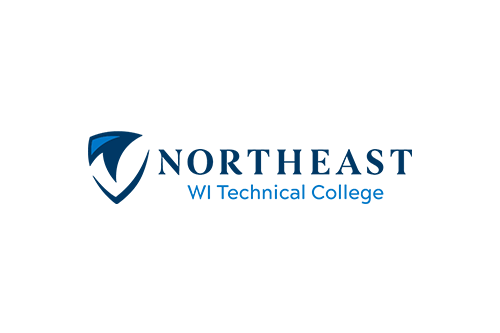# Mathematics and Logic

Course Number: 10804133
Credits: 3.00

## Course Description

10-804-133 MATH & LOGIC ...Students will apply problem solving techniques from discrete mathematics.Topics include symbolic logic, sets, algebra and base number systems (Prerequisite: Next Gen Arith score greater/equal to 250 AND Rdg score greater/equal to 250; OR TABE A 9/10 Math greater/equal to 12.7 AND Rdg greater/equal to 8.8; OR TABE 11/12 Math greater/equal to 780 AND Rdg greater/equal to 573; OR ACT Math score greater/equal to 15 AND ACT Rdg score greater/equal to 16; OR prep courses-contact academic advisor 920-498-5444).

• Summer
• Fall
• Spring

## Course Competencies

1. Solve applied algebraic problems
2. Utilize heuristic tools for problem solving
3. Convert between place value number systems
4. Apply number systems to problem solving
5. Apply principles of set theory
6. Apply symbolic logic principles